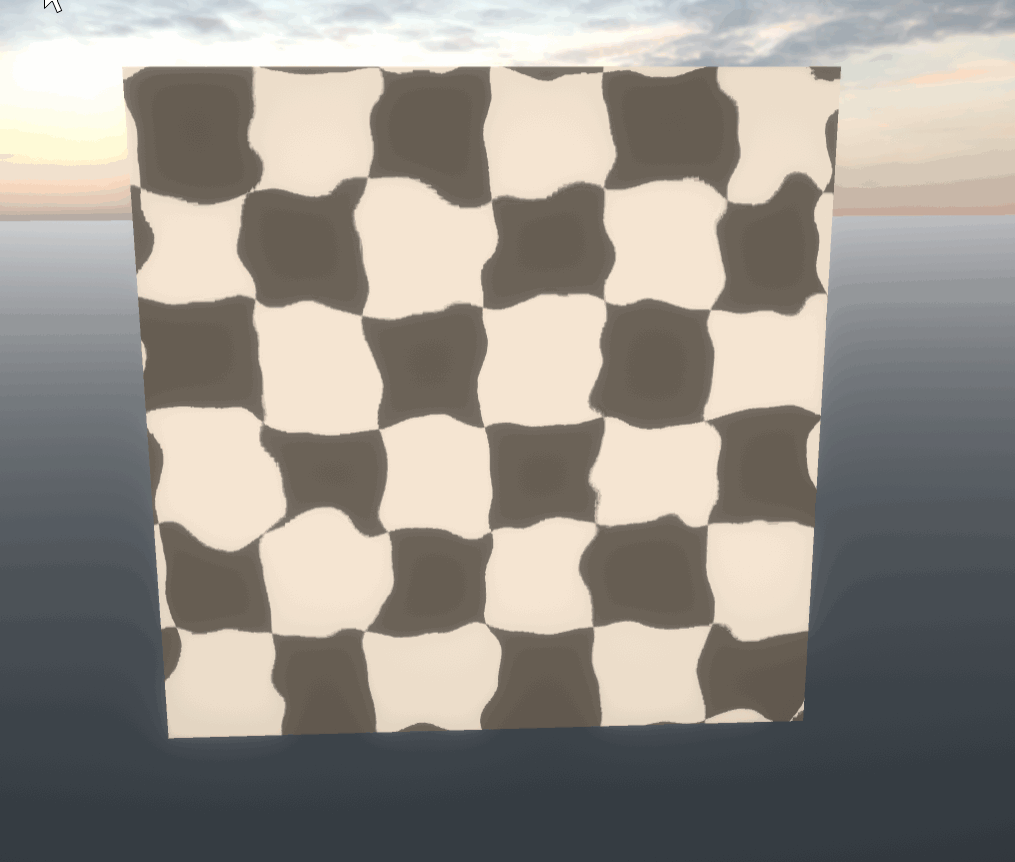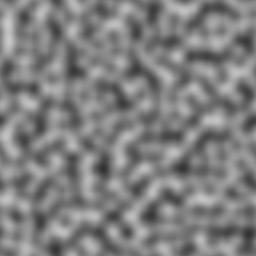top of page
Search
• alexlpc2015

# [Shader Notes] #03 - Distortion

Hi and welcome to my Shader Notes Series!

This is a comprehensive project with notes about shaders of nearly every common effect included (implemented in Unity). I will explain the key points and theories behind them for you to understand them thoroughly and then enjoy creating your own shaders with proficiency.

Let's start learning together!

## #03 - Distortion

Difficulty: 2/10

Points covered:

• UV displacement by noise texture

Distortion effect is a common effect often used to simulate heatwave, water, smoke, etc.

## Final effect:### Setup:

Create a new material and attach it to an object.

Prepare the properties and declare them in CGPROGRAM.

```//Properties
_MainTex ("Texture", 2D) = "white" {}
_DisplacementTex("Displacement Texture", 2D) = "white" {}
_Magnitude("Magnitude", Range(0, 1)) =  0.5
_Speed("Speed", float) =  1.0

```

In the vertex shader, we need to prepare both the uv coordinates for the main texture and the noise texture. Do not forget to declare the _ST variable for the TRANSFORM_TEX macro.

```sampler2D _MainTex;
float4 _MainTex_ST;
sampler2D _DisplacementTex;
float4 _DisplacementTex_ST;

//...

v2f vert (appdata v)
{
v2f o;
o.vertex = UnityObjectToClipPos(v.vertex);
o.uv = TRANSFORM_TEX(v.uv, _MainTex);
o.duv = TRANSFORM_TEX(v.uv, _DisplacementTex);
return o;
}

```

### Point #1: UV Displacement

Recall that we can produce seemingly random effects by sampling a noise texture (or they can be procedurally generated, which will be discussed in other notes):First, calculate the displacement value for the x and y components of the texture coordinate. Notice that i.duv is incremented by a sine function with _Time inside. This will create a smooth, cycling flow of output. We then use the edited uv to sample the noise texture to get the displacement value.

```float2 disp = tex2D(_DisplacementTex, i.duv+ sin(_Time.y * _Speed * 0.05)).xy;

```

Since the displacement value we sampled from an image ranges from (0,1), we need to project the range to (-1,1) to get a all-direction effect. This is done by multiplying it by 2 and then minus 1.

```disp = ((disp * 2) - 1) * _Magnitude;

```

Finally, add the displacement value to the original uv and sample the main texture.

```float4 col = tex2D(_MainTex, i.uv + disp);
return col;

```

And it's done!

We will look at an application of this effect in the next shader note. See you there!

```Shader "AlexLiu/Distortion_0"
{
Properties
{
_MainTex ("Texture", 2D) = "white" {}
_DisplacementTex("Displacement Texture", 2D) = "white" {}
_Magnitude("Magnitude", Range(0, 1)) =  0.5
_Speed("Speed", float) =  1.0
}
{
Tags { "RenderType"="Opaque" }
Cull Off

Pass
{
CGPROGRAM
#pragma vertex vert
#pragma fragment frag

#include "UnityCG.cginc"

struct appdata
{
float4 vertex : POSITION;
float2 uv : TEXCOORD0;
};

struct v2f
{
float2 uv : TEXCOORD0;
float2 duv : TEXCOORD1;
float4 vertex : SV_POSITION;
};

sampler2D _MainTex;
float4 _MainTex_ST;
sampler2D _DisplacementTex;
float4 _DisplacementTex_ST;
float _Magnitude;
float _Speed;

v2f vert (appdata v)
{
v2f o;
o.vertex = UnityObjectToClipPos(v.vertex);
o.uv = TRANSFORM_TEX(v.uv, _MainTex);
o.duv = TRANSFORM_TEX(v.uv, _DisplacementTex);
return o;
}

fixed4 frag (v2f i) : SV_Target
{
float2 disp = tex2D(_DisplacementTex, i.duv+ sin(_Time.y * _Speed * 0.05)).xy ;
disp = ((disp * 2) - 1) * _Magnitude;
float4 col = tex2D(_MainTex, i.uv + disp);
return col;
}
ENDCG
}
}
}

```